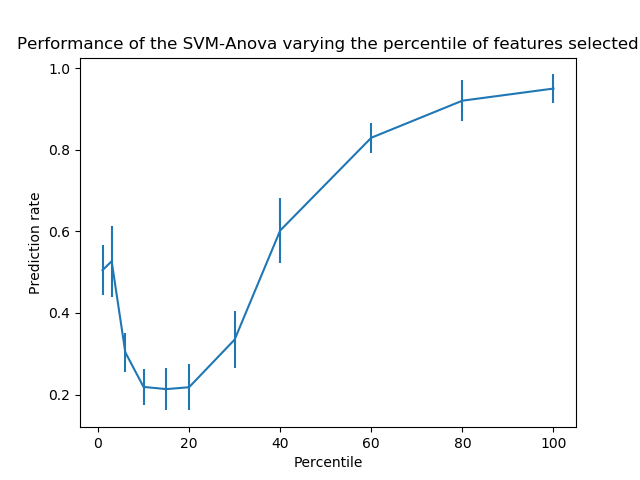/scikit-learn

# SVM-Anova: SVM with univariate feature selection

This example shows how to perform univariate feature selection before running a SVC (support vector classifier) to improve the classification scores.```print(__doc__)

import numpy as np
import matplotlib.pyplot as plt
from sklearn.feature_selection import SelectPercentile, chi2
from sklearn.model_selection import cross_val_score
from sklearn.pipeline import Pipeline
from sklearn.svm import SVC

# #############################################################################
# Import some data to play with
# Throw away data, to be in the curse of dimension settings
X = X[:200]
y = y[:200]
n_samples = len(y)
X = X.reshape((n_samples, -1))
X = np.hstack((X, 2 * np.random.random((n_samples, 200))))

# #############################################################################
# Create a feature-selection transform and an instance of SVM that we
# combine together to have an full-blown estimator

transform = SelectPercentile(chi2)

clf = Pipeline([('anova', transform), ('svc', SVC(gamma="auto"))])

# #############################################################################
# Plot the cross-validation score as a function of percentile of features
score_means = list()
score_stds = list()
percentiles = (1, 3, 6, 10, 15, 20, 30, 40, 60, 80, 100)

for percentile in percentiles:
clf.set_params(anova__percentile=percentile)
# Compute cross-validation score using 1 CPU
this_scores = cross_val_score(clf, X, y, cv=5, n_jobs=1)
score_means.append(this_scores.mean())
score_stds.append(this_scores.std())

plt.errorbar(percentiles, score_means, np.array(score_stds))

plt.title(
'Performance of the SVM-Anova varying the percentile of features selected')
plt.xlabel('Percentile')
plt.ylabel('Prediction rate')

plt.axis('tight')
plt.show()
```

Total running time of the script: ( 0 minutes 1.323 seconds)

Gallery generated by Sphinx-Gallery